From 09:00 PM Friday, August 7 - 10:30 AM CST Saturday, August 8, ni.com will be undergoing system upgrades that may result in temporary service interruption.

We appreciate your patience as we improve our online experience.

# Sources of Error in IQ Based RF Signal Generation

Publish Date: Nov 29, 2016 | 21 Ratings | 4.00 out of 5 | Print | Submit your review

## Overview

The following document decribes the basic architecture of IQ-based RF signal generators and provides an in-depth analysis of ways to characterize types error in communicaitons systems.

### Introduction

This tutorial is part of the National Instruments Signal Generator Fundamentals. Each tutorial in this series will teach basic concepts about the architecture, features, or strategies for baseband IQ signal generation.

With each new communications protocol, engineers are finding ways to more efficiently use a given bandwidth than ever before. WiFI, for example, has evolved to use better spectral efficiency with new methods such as OFDM and now MIMO. In addition, new communications standards like WiMAX have successfully improved channel throughput through higher-order modulation schemes such as 256-QAM. However, while advances in spectral efficiency have enabled some technologies to achieve higher throughput, today’s systems are often limited by various characteristics of the physical hardware. Thus, understanding the sources of error in these systems becomes paramount. One of the most popular architectures for signal generation is in-phase and quadrature-phase (IQ) signal generation. This white paper explains several common sources of error on IQ-based signal generation including gain imbalance, skew, offset.  It also explores the affects of higher order modulation schemes such as 256-QAM, which is used in WiMAX.

### 1. Communications System Architectures

Modern digital communications systems require significant digital signal processing and sophisticated analog circuitry.  On a high level, we can represent a communications system with several basic stages: from the bitstream to the physical channel. This is illustrated in the diagram below: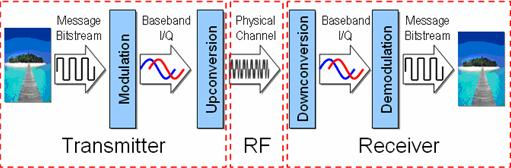Figure 1. Block Diagram of Communications System

As the block diagram above illustrates, communications signals require several stages of signal processing, both in the digital domain and analog domain.  To start with, let us expand our diagram above to example a typical RF transmitter, shown below: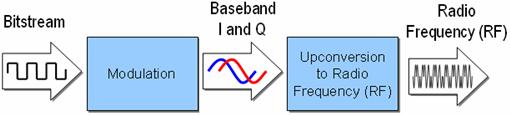Figure 2. Components of an RF Transmitter

In an IQ-Based RF transmitter, digital signal processing (DSP) chips can perform modulation, interpolation, and pulse-shaped filtering to a digital baseband signal.  Because the signal processing is performed digitally, the error is minimal.  However, once the communications signal is implemented in the analog domain with a digital-to-analog converter, it is subject to many sources of analog impairments. Below we illustrate a typical transmitter architecture on a component level.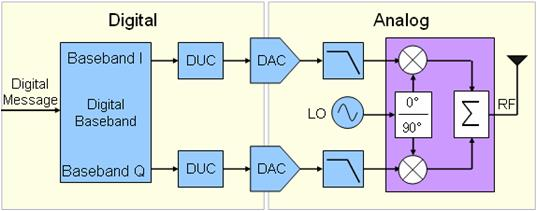Figure 3. Block Diagram of Direct Upconversion RF Transmitter

As the diagram above illustrates, a typical transmitter is required to perform signal processing both to digital signals and analog signals.  On the analog side, a direct upconverter requires the generation and mixing of two analog baseband signals with a local oscillator (LO). As one might suspect, the translation of a digital message signal into digitally upconverted I and Q signals can be performed with little or no error. However, once digital baseband signals enter the analog domain through digital-to-analog conversion, they are prone to a variety of sources of error. In this document, we will evaluate each of the various sources of error and the effect of that error on the RF signal. The specific error sources evaluated include frequency response of the digital-to-analog converter (DAC), baseband synchronization (quadrature skew), IQ gain imbalance, and phase noise. In addition, the effect of each of these errors depends, at least in part, on the mechanism of upconversion or downconversion that is used in the system. Typical approaches to upconversion and downconversion are direct upconversion (shown in figure 3), and heterodyne (intermediate frequency) upconversion, discussed in a later section.

### 2. Direct (Homodyne) Upconversion

Homodyne or direct upconversion, one of the simplest upconversion techniques, is common in many wireless devices.  It is often preferred because of its simplicity and cost effectiveness.  In a homodyne upconversion implementation, analog I and Q signals are mixed with respective in-phase and quadrature-phase versions (ninety degrees out of phase) of a local oscillator (LO). The local oscillator is the carrier frequency of the RF signal. Finally, the translated I and Q components are summed (or subtracted) to produce the final RF signal.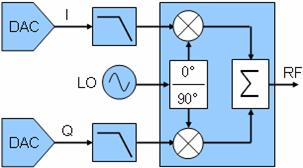Figure 4. Hardware Diagram of Direct Upconversion

In the frequency domain, direct upconversion translates baseband I and Q signals centered at a zero frequency to an RF frequency.  The frequency domain of these signals is shown below: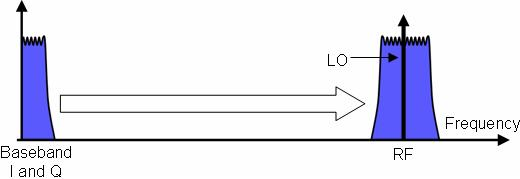Figure 5. Frequency Domain of Direct Upconversion to RF

As Figure 5 illustrates, direct upconversion mixes each baseband signal with an LO at the center frequency of the RF signal. In addition, Figure 5 also illustrates the bandwidth relationship between each baseband signal and the RF input. As the figure illustrates, each baseband I and Q signal is half the bandwidth of the RF signal. Thus, by using two DACs in a direct frequency translation approach, the maximum modulation bandwidth for the RF signal is exactly twice the bandwidth of each DAC.

Direct downconversion uses an architecture that is the reverse of direct upconversion. A typical hardware implementation of a direct downconverter is shown below.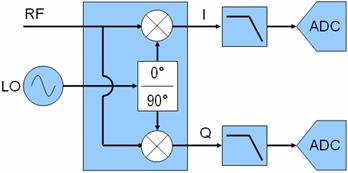Figure 6. Hardware Diagram of Direct Downconversion

As the figure illustrates, a direct downconverter uses two analog signal mixers to mix the RF signal with an in-phase and quadrature-phase version of the LO. These mixed signals are the baseband I and Q signals, which are filtered and then digitized with an analog-to-digital converter (ADC). Because the RF signal is mixed with two oscillators that are exactly 90 deg out of phase, the digitized baseband I and Q signals provide the receiver with an estimation of the phase and amplitude of the RF signal.

### 3. IF (Heterodyne Upconversion)

A second approach to upconversion and downconversion is the heterodyne method, which uses an intermediate frequency or IF. With this method, the baseband I and Q signals first undergo direct upconversion to an intermediate frequency. They are then mixed with an addition local oscillator to reach the RF frequency. A typical transmitter using the heterodyne approach to upconversion is illustrated below: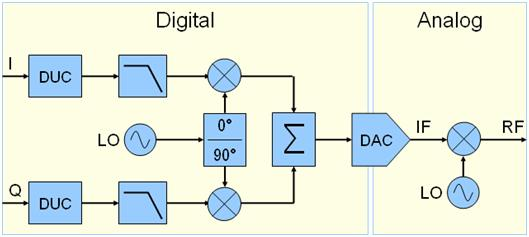Figure 7. Block Diagram of Homodyne Upconverter

As the figure illustrates, heterodyne (IF) upconversion is a slightly more complex method of upconversion. However, the figure also illustrates that direct upconversion can typically be done digitally. In deployed IF upconverters, digital upconversion to the IF typically occurs in a DSP or FPGA. The IF waveform is then generated by a single digital-to-analog converter (DAC) before being upconverted to the RF signal. Typical intermediate frequencies range between 15 and 170 MHz and at higher frequencies, require a high-bandwidth DAC. In addition, the maximum RF bandwidth using IF upconversion depends upon the IF frequency. For example, for an IF of 15 MHz, the maximum RF bandwidth is 30 MHz and requires a DAC with 30 MHz of bandwidth or greater. The frequency domain of analog upconversion is illustrated below.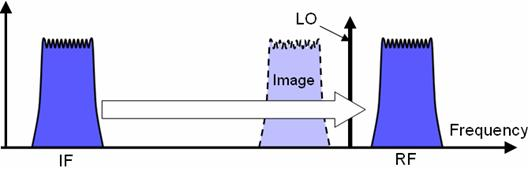Figure 8. Frequency Domain of Heterodyne Upconversion to RF

As the Figure 8 illustrates, the LO for a single-stage IF upconverter should be at the frequency difference of the RF carrier and the intermediate frequency. In addition, the mixing process also produces an image at the frequency difference of the LO and the intermediate frequency. Thus, for devices using this method, filters must be used to ensure that the image does not affect adjacent channels. An alternative is to use a higher frequency IF to ensure that the image is farther removed from the desired RF signal.

In some instances, a series of mixers are used with multiple filters to remove these signal images. For example, in the NI PXI-5671 vector signal generator, several mixers and filters are used in conjunction to eliminate IF images.

As one might expect, the sources of error in heterodyne upconversion are slightly different than from the direct upconversion approach. Because heterodyne upconversion uses digital signal processing to directly upconvert baseband I and Q waveforms to the IF, they are immune to the phase and gain errors associated with analog signal mixing. However they typically require wider bandwidth DACs, which are more susceptible to errors such as passband flatness and harmonic distortion. In addition, implementation of filters and multiple stages of LOs to eliminate IF spectral images requires sophisticated circuitry. Thus, for most commercial applications, direct upconversion is the most common approach because of its simplicity and cost-effectiveness.

### 4. Characterizing and System Error

As mentioned previously mentioned, direct upconversion is subject to a several sources of error because of part-to-part variation and non-linearity of analog components. In this section, we will evaluate the affects of DC offsets, phase noise, quadrature skew, and gain imbalance on the performance of a communications system. These are illustrated in the Figure 9.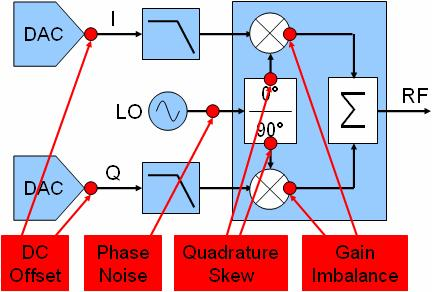Figure 9. Common Sources of Error in a Direct Upconversion Transmitter

Communications systems require accurate transitions in phase, amplitude, and frequency of a modulated RF signal.  In fact, errors due to DC offset, phase noise, quadrature skew, or gain imbalance each impair the accuracy of the signal generated.  Because the accuracy of each signal transition determines factors such as bit error rate (BER) and order of modulation scheme (4-QAM versus 256-QAM), they have a direct affect on the potential system throughput.

With the LabVIEW Modulation Toolkit, you can model each of these system impairments to evaluate their effects on system performance. Thus, to analyze the effects of each of these impairments, you can characterize performance with two types of measurements – modulation measurements and single tone/spectral measurements.

### 5. Modulation Measurement Error Characterization

When characterizing modulation measurements, it is important to note that the phase, amplitude, and frequency of the RF signal translated to the amplitude of each I and Q vector. Thus, errors in the baseband signal translate to errors in the RF signal.

As a point of reference, the graph below illustrates two time-domain baseband signals, I and Q (I in red, Q in blue). When recovering actual symbols, I and Q must be precisely synchronized and sampled at appropriate intervals. In the time-domain graph below, the ideal symbol locations for the given baseband signals are illustrated by the large dots.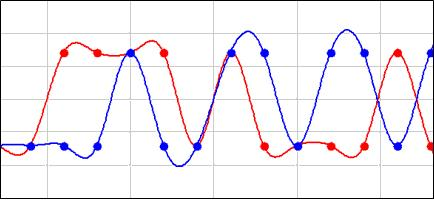Figure 10. Time Domain of a Baseband Signal

Thus, the recovery of actual symbols depends upon various characteristics of the IQ symbol.  These characteristics include accuracy of the amplitude, phase, and frequency of each baseband signal.  For simple modulation schemes such as 4-QAM or BPSK, relatively inaccurate baseband signals are tolerable.  However, for higher order modulation schemes such as 64-QAM and 256-QAM, baseband accuracy is essential.  For these modulation schemes, small errors of DC offset, phase noise, quadrature skew, or IQ gain imbalance can make the transitions of the RF signal too difficult to distinguish.  In these situations, the receiver is unable to accurately assess the relative phase and frequency of the baseband waveform and the constellation plot will begin to spin.  For example, consider several cases of baseband signals with significant error.

First, consider the scenario where both baseband I and Q signals experience a 10% DC offset.  For a 4-QAM modulation scheme, the DC error is insignificant and the signal can be successfully demodulated.  However, for a 64-QAM modulation scheme, the error is significant enough to prevent carrier recovery.  Thus, the constellation plot begins to spin when the demodulation algorithm is unable to accurately estimate the phase or frequency of the baseband signal.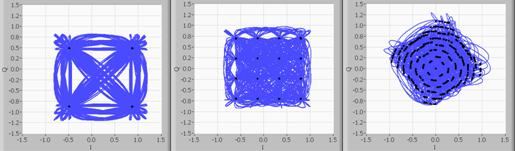Figure 11. DC Offset in 4-QAM, 16-QAM, and 64-QAM Signals

In addition, a similar affect can be observed when phase noise is present in the LO.  Phase noise creates instantaneous frequency error of the baseband signal.  For higher order modulation schemes, phase noise also prevents carrier recovery and causes the constellation plot to spin.  For the examples below, we illustrate the constellation plots of two modulation schemes when a phase noise of -50 dBc at a 1 KHz offset is observed.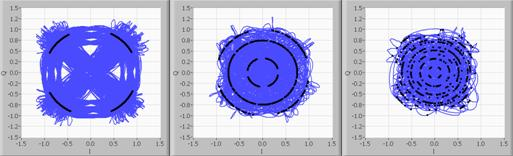Figure 12. Phase Noise in 4-QAM, 16-QAM, and 64-QAM Signals

Next we evaluate quadrature skew as source of error.  This source of error is typically observed in the LO splitter, which divides the LO into an in-phase and a quadrature-phase signals.  While an ideal system would result in each of these being exactly 90 degrees out of phase, some systems specify up to 3 degrees or more of skew.  For lower order modulation schemes, quadrature skew has relatively little affect on system throughput.  However, like other sources of error, higher order modulation schemes such as 64-QAM are significantly impaired.  Below, we contrast the performance of two systems with a quadrature skew of 16%.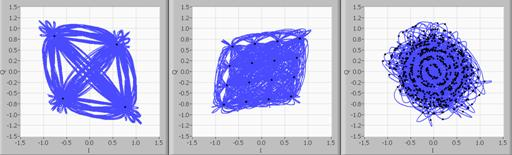Figure 13.  Quadrature Skew in 4-QAM, 16-QAM, and 64-QAM Signals

Finally, we evaluate the affects of IQ gain imbalance.  This source of error is caused by amplitude errors in the DAC’s or because of inconsistencies between each of the analog mixers.  Again, for higher order modulation schemes such as 64-QAM IQ gain imbalance of even a few dB can prevent proper demodulation of the signal.  This is illustrated in the figures below: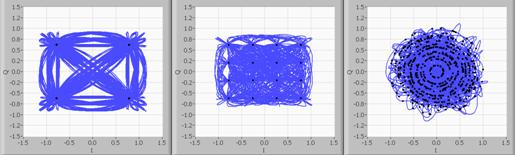Figure 14. IQ Gain Imbalance in 4-QAM, 16-QAM, and 64-QAM Signals

Thus, as the figures above illustrate, we can error in each component of a direct upconverter results has a significant affect on the ability of a receiver to successfully demodulate a signal.  Thus, when characterizing an RFIC, it is important to characterize overall performance with some of the measurements shown above.

### 6. Spectral Measurement Error Characterization

A second method that can be used to characterize DC, gain, or phase error in an IQ-based RF transmitter is by generating single tones at 90 deg of phase skew into the and Q inputs. In general, upconversion subsystem, which contains two mixers and a LO conversion, can cause significant error. One way test this subsystem is characterize the carrier and sideband (or image) suppression of the system, as illustrated in the diagram below.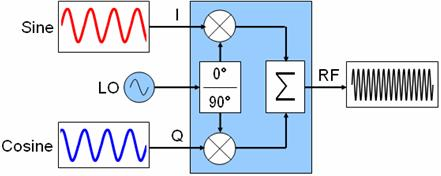Figure 15. Characterization of Direct Quadrature Modulator

As the figure illustrates, both I and Q inputs are supplied with single tones that are 90 deg out of phase. Moreover, in an ideal system without DC offset, quadrature skew, or gain imbalance, the result should be a single tone at a frequency of LO + sine (or cosine, because they are the same frequency).

While this can be proved mathematically, it is sufficient to represent this graphically as well in the following diagram.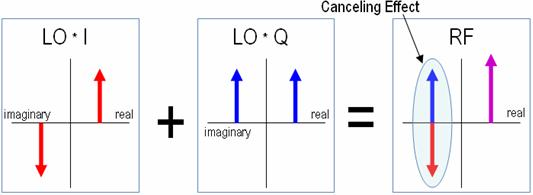Figure 16. Graphical Representation of Image Cancellation

As the diagram above illustrates, mixing a sine tone with the LO will create two images at frequencies of LO – I or Q and LO + I or Q.  In addition, this occurs for both mixers and each I and Q baseband signal.  However, because I and Q are ninety degrees out of phase, and because the in-phase and quadrature-phase LO’s are ninety degrees out of phase, an ideal system will result in two signals at LO – I or Q that are perfectly 180 degrees out of phase.  Thus, when these signals are added together, the LO – I or Q signals should cancel each other out, leaving a single tone at LO + I or Q.  In a real system, errors such as quadrature skew and IQ gain imbalance will prevent the LO – I or Q from completely cancelling.  As a result, we are left with an unsuppressed sideband, also known as an unsuppressed image.  In addition, any DC offset in either I or Q inputs will create an additional tone at the exact frequency of the LO.  This is referred to as an unsuppressed carrier.  Both of these tones are frequently expressed in dBc, which is the amplitude difference between the tone and the LO.  This is illustrated in the graph below: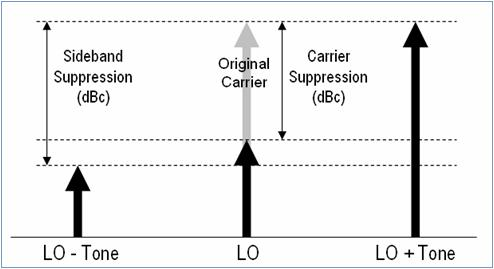Figure 17. Measuring Sideband and Carrier Suppression

As the figure above illustrates, the sideband and carrier suppression are measured in dBc, relative to the original carrier.

### 7. Generating Impaired Baseband Signals

In addition to evaluating system error in a communications system, you can also generate baseband signals that can either simulate system error or compensate for it. Adjustments to baseband I and Q signals typically involve tweaking the gain, phase, or amplitude. In this section, we describe two scenarios where applying adjustments to the baseband signal is necessary.

### Simulating Subsystem Error with Baseband Adjustments

In a typical RF transmitter implementation, individual components, including the digital-to-analog-converter are subject to slight errors in gain and DC offset.  Thus, when considering a DAC or direct quadrature modulator, it is important to simulate the error of the DAC signals by applying gain or DC offset adjustments to the baseband I or Q signals.  Error in DAC output is illustrated by the diagram below: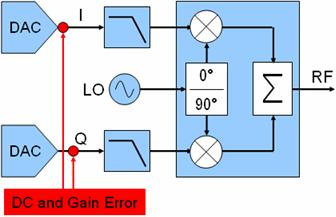Figure 18. Simulating DAC DC and Gain Error

As mentioned in a previous section, errors in DC offset or gain of each baseband input to a direct quadrature modulator results in an unsuppressed carrier and sideband. In addition, these errors affect the quality of the modulated signal. Thus, in order to verify whether a given subsystem, such as a direct quadrature modulator, is capable of use with a particular standard such as WiMAX, the device can be tested using baseband inputs that have been adjusted to simulate the accuracy of the baseband DACs.  These adjustments can typically be performed in software by creating an impaired waveform in LabVIEW, or in hardware by applying DC or gain impairments to the arbitrary waveform generator.

### Compensating for Subsystem Error with Baseband Adjustments

A second instance where it is important to apply baseband adjustments is when configuring systems to generate wideband RF signals.  With these systems, a benchtop vector signal generator (VSG) can be used to generate wideband RF signals using external baseband I and Q inputs.  An implementation of a typical system is shown below: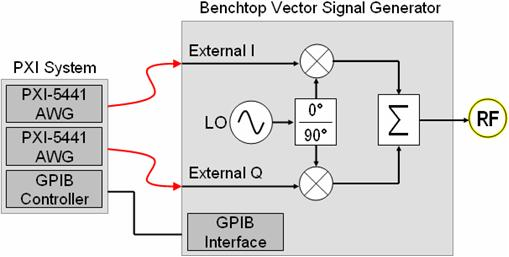Figure 19. Configuration of a Hybrid PXI / Benchtop Wideband RF Generator

As the figure illustrates, two PXI arbitrary waveform generators (AWGs) are synchronized to generate I and Q signals for the external baseband input to the benchtop vector signal generator. Using this approach, you can compensate for errors in the vector signal generator. By adjusting AWG characteristics such as DC offset, gain, and skew, you can compensate for the gain and phase errors of the vector signal generator. As a result, you can generate the most accurate signal possible.

As an example, the following sideband and carrier suppression measurements were made using an Agilent E443x series VSG with two PXI-5441 arbitrary waveform generators. By adjusting the DC offset, gain, and phase of the arbitrary waveform generators, you can compensate for the VSG to generate the most accurate RF signal possible. This is illustrated below: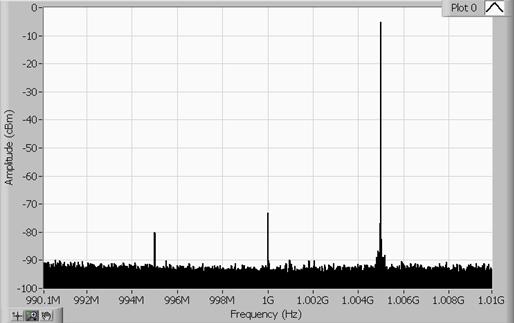Figure 20. Sideband and Carrier Suppression of RF Signal with Adjusted Baseband

As the image above illustrates, generation of a 1GHz RF signal at -5 dBm can be done with better than -75 dBc of sideband suppression and -65 dBc of carrier suppression by manually tweaking the baseband signal.  Thus, for test cases which require highly accurate RF signals, small adjustments of the baseband signal can be implemented to improve the accuracy of the RF signal.

### Summary

As communications systems continue to evolve, the affects of error in IQ-based signal generation continues to become more prevalent. For example, while 3 deg of quadrature skew might be acceptable for a cell phone using MSK modulation, it is entirely unacceptable for a WiMAX transceiver using 256-QAM as a modulation scheme. Thus it is important to understand and characterize system error in communications transceivers. As a result, modulation and spectral measurements are an important part of system characterization.

### Related Products

NI modular instruments can be used with the LabVIEW modulation toolkit to implement a complete communications sytem, from baseband waveforms to RF signals.  In addition, these products can test devices used in both homodyne and heterodyne communications systems.  As an example, common NI products include the following:

• PXI-5441, 100 MS/s Arbitrary Waveform Generator with Onboard Signal Processing
• PXI-5671, 2.7 GHz Vector Signal Generator with Digital Upconversion
• PXI-5661, 2.7 GHz Vector Signal Analyzer with Digital Downconversion
• PXI-5142, 100 MS/s Digitizer with Onboard Signal Processing

The IF digitizer can be used to acquire both intermediate frequency and baseband signals.  In addition, OSP enables IF signals to be digital downconverted to baseband.  For baseband acquisition, OSP enables decimation of baseband waveforms to the appropriate symbol rate.

### Ratings

Rate this document